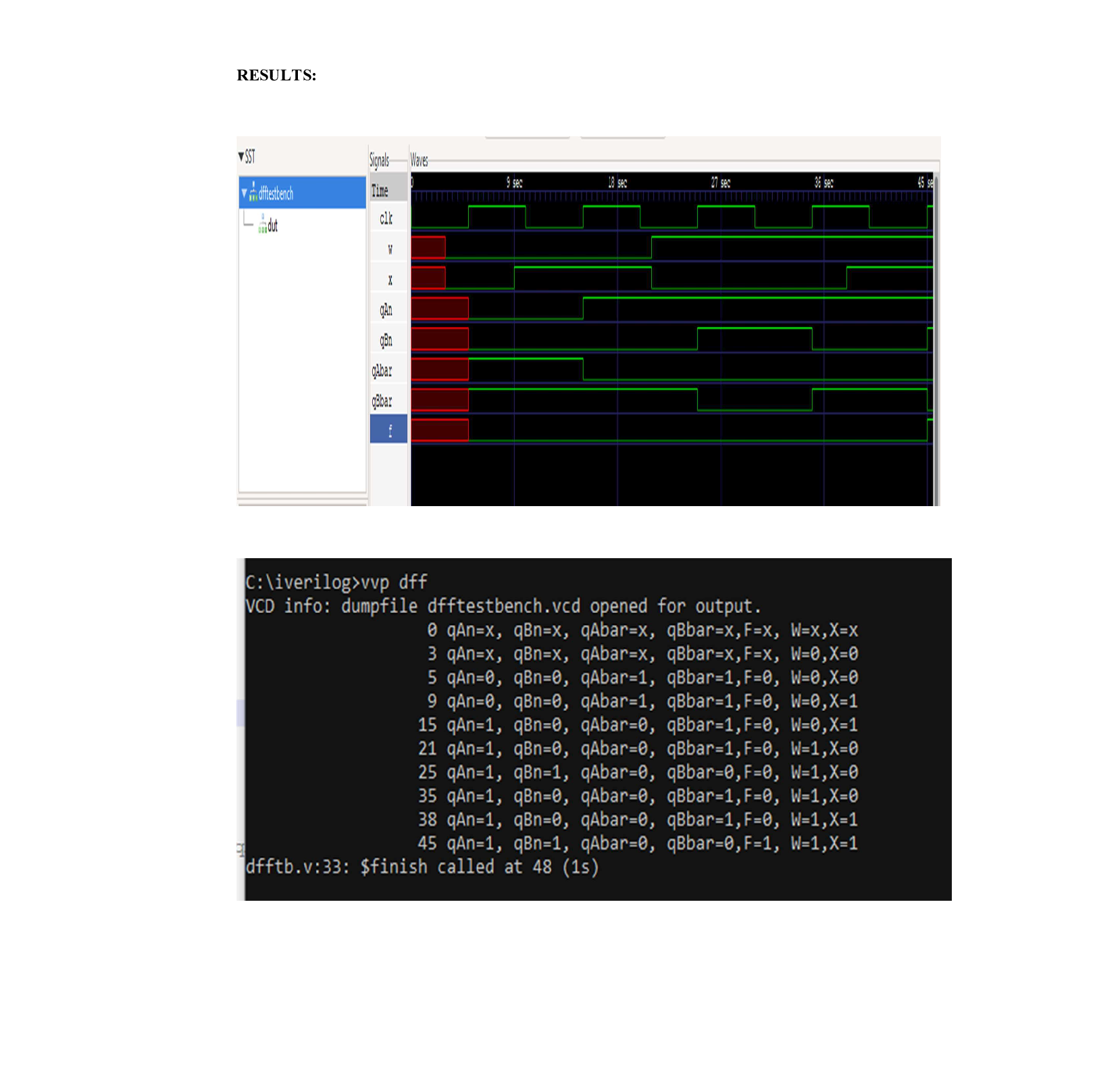Question

Analog Electronics

5. Develop a Verilog HDL design of the circuit provided in problem #1. Show your HDL code as well as the simulation results.Verified### Question 41990Analog Electronics

The exponential Fourier series for someperiodic signal is given by
v(t)=\sum_{n=-\infty}^{\infty} \frac{5|n|}{n^{2}+1} \cdot e^{j 2 \pi 6000 n t}
n=-00if this signal is passed through a band-pass filter with cutoff frequencies fa =10000
\mathrm{HZ} \text { and } f_{C U}=20000 \mathrm{HZ} \text {. }
Plot the spectrum of the input signal from -20000 HZ to 20000 HZ.
b. Write down the equation for the output signal and plot the spectrum of the output signal.
c. How much power is contained in the input signal between -20000 HZand 20000 HZ and how much power is contained in the output signal?
d. What is the output signal bandwidth?

### Question 41989Analog Electronics

2. A periodic signal has the following Fourier series representation
v(t)=\sum_{n=1}^{\infty} \frac{3}{2 n-1} \cos 2 \pi n 3000 t \text { is passed through an ideal low-pass filter with }
cutoff frequency of 11 KHZ.
Plot the spectrum of the input signal up to 20 KHZ.
b. Plot the spectrum of the output signal.
с.What is the output signal bandwidth?
d. Calculate the power contained in the output signal.

### Question 41988Analog Electronics

1. Find the Fourier transform and plot the spectrum of the following signal
v(t)=4 \cos 2 \pi 5000 t \cdot \sin 2 \pi 10000 t

### Question 41198Analog Electronics

2. (5 pts.) What is Nyquist's theorem? What problem do we encounter if we do not meet Nyquist's sampling rate?

### Question 41197Analog Electronics

### Question 41196Analog Electronics

Sketch the successive approximation ADC circuit and explain how it works.

### Question 41195Analog Electronics

(5 pts.) Given an analog output signal whose voltage should range from 0 to 10V, and an 8-bitdigital encoding, provide the encodings for the following desired voltages: (a) OV, (b) 1V, (c) 5.33V,(d) 10V, what is the resolution of the conversion?

### Question 41194Analog Electronics

-) Briefly describe the three main steps implemented in an Analog-to-Digital Converter.

### Question 38988Analog Electronics

Now calculate the value of R2 given that the bias point is 1V, Supply Voltages 20V and the total resistance R1+R2 is 100k2ohms

### Question 38987Analog Electronics

9)lf the circuit is required to have a gain of 30dBs and Rc=10KN, calculate the value of Re

### Submit query